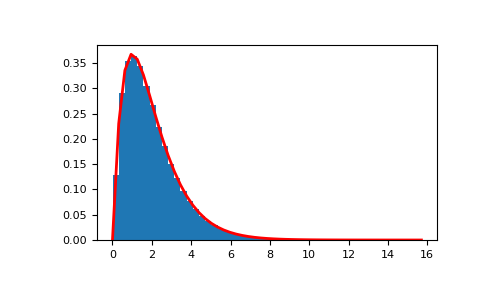# numpy.random.Generator.standard_gamma¶

method

Generator.standard_gamma(shape, size=None, dtype='d', out=None)

Draw samples from a standard Gamma distribution.

Samples are drawn from a Gamma distribution with specified parameters, shape (sometimes designated “k”) and scale=1.

Parameters
shapefloat or array_like of floats

Parameter, must be non-negative.

sizeint or tuple of ints, optional

Output shape. If the given shape is, e.g., (m, n, k), then m * n * k samples are drawn. If size is None (default), a single value is returned if shape is a scalar. Otherwise, np.array(shape).size samples are drawn.

dtype{str, dtype}, optional

Desired dtype of the result, either ‘d’ (or ‘float64’) or ‘f’ (or ‘float32’). All dtypes are determined by their name. The default value is ‘d’.

outndarray, optional

Alternative output array in which to place the result. If size is not None, it must have the same shape as the provided size and must match the type of the output values.

Returns
outndarray or scalar

Drawn samples from the parameterized standard gamma distribution.

scipy.stats.gamma

probability density function, distribution or cumulative density function, etc.

Notes

The probability density for the Gamma distribution iswhereis the shape andthe scale, andis the Gamma function.

The Gamma distribution is often used to model the times to failure of electronic components, and arises naturally in processes for which the waiting times between Poisson distributed events are relevant.

References

1

Weisstein, Eric W. “Gamma Distribution.” From MathWorld–A Wolfram Web Resource. http://mathworld.wolfram.com/GammaDistribution.html

2

Wikipedia, “Gamma distribution”, https://en.wikipedia.org/wiki/Gamma_distribution

Examples

Draw samples from the distribution:

>>> shape, scale = 2., 1. # mean and width
>>> s = np.random.default_rng().standard_gamma(shape, 1000000)


Display the histogram of the samples, along with the probability density function:

>>> import matplotlib.pyplot as plt
>>> import scipy.special as sps
>>> count, bins, ignored = plt.hist(s, 50, density=True)
>>> y = bins**(shape-1) * ((np.exp(-bins/scale))/
...                       (sps.gamma(shape) * scale**shape))
>>> plt.plot(bins, y, linewidth=2, color='r')
>>> plt.show()#### Previous topic

numpy.random.Generator.standard_exponential

#### Next topic

numpy.random.Generator.standard_normal